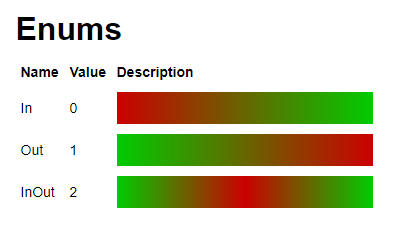It would be cool to see more easing styles added. The current ones are: Linear, Sine, Back, Quad, Quart, Quint, Bounce, and Elastic.

With the release of the TweenService, it would be awesome if additional easing styles were added to give more options for developers.

Here are my proposed additions, which I have been using for many years from Penner’s easing functions in Lua:

The easings.net website is good for visualizing these.

If anyone else has any easing styles that they know of, feel free to pitch them too.

38 Likes

I was literally about to make a similar post like this, support!

A generic [0, 1] -> [0, 1] easing function parameter would be nice.

What do you mean? Isn’t that what the Linear style is for?

I think he means the ability to provide a function as easing style instead of picking a hardcoded one.

3 Likes

That’s feasible but it wouldn’t benefit from C++ acceleration…

The point isn’t about making it faster, but rather giving more options for developers to use natively with the TweenService. Perhaps what @MrHistory said would be an option for this. But if we went that route, then I’d say it would also be cool to allow the TweenService to spit out the raw output too, and allow us to bind a callback to it or something…but that’s a whole separate feature request of it’s own.

Is the acceleration even necessary? Afaik the easing function is only being called once per frame.

If performance was even a problem inputs could be rounded and results could be cached on the C++ end.

Every animation in my game uses easing styles implemented in lua, and it works extremely well:

My code lets me pass arbitrary functions to my animation system, but there are a few styles I use often. The roblox engine overcomplicates easing styles, all they need to be is a method that transforms values in the unit interval. This is what my lerp module looks like:

``````local cos = math.cos
local sin = math.sin

local pi = math.pi
local pi2 = pi * 2
local hpi = pi / 2

return {
--[[ Interpolation Transformations, aka "XForms"
Used for transforming unit values
Input Domain: [0, 1] -- unit interval
Output Range: [0, 1] -- unit interval
f(0) = 0 -- (might not be exact for sin/cos)
f(1) = 1 -- (might not be exact for sin/cos)
--]]
lin = function(x) -- Linear, Slopes: {1}
return x
end;

--[[ I named these years ago
sinda = union{0,0, "sind", 0.5,0.5, "sina", 1,1}
--]]
sina = function(x) -- Sine quadrant 1, Slopes: {1, 0}
return sin(hpi * x)
end;
sind = function(x) -- Sine quadrant 4, Slopes: {0, 1}
return 1 - cos(hpi * x)
end;
sinda = function(x) -- Sine quadrants 4 and 1, Slopes: {0, 1, 0}
return 0.5 - cos(pi*x) * 0.5 -- Perfectly smooth
end;

sstep = function(x) -- 3rd order smoothstep
return x * x * (3 - 2 * x) --3*x^2 - 2*x^3
end;
sstep2 = function(x) -- 5th order smoothstep
return x * x * x * (x * (6 * x - 15) + 10) --6*x^5 - 15*x^4 + 10*x^3
end;
sstep3 = function(x) -- 7th order smoothstep
return -20*x^7 + 70*x^6 - 84*x^5 + 35*x^4 -- o_O
end;

bounce = function(x) -- Should be equal to the roblox "Bounce" easing style
return
x < 1/2.75   and x*x * 7.5625 or
x < 2/2.75   and (x-1.5/2.75)*(x-1.5/2.75) * 7.5625 + 0.75 or
x < 2.5/2.75 and (x-2.25/2.75)*(x-2.25/2.75) * 7.5625 + 0.9375 or
(x-2.625/2.75)*(x-2.625/2.75) * 7.5625 + 0.984375
end;
elastic = function(x) -- Should be equal to the roblox "Elastic" easing style
return 1 + 2^(-20*x) * sin((x*2 - 0.3/4) * (pi2/0.3));
end;

-- Static
const_0 = function()
return 0
end;
const_1 = function()
return 1
end;

snap_0_1 = function(x)
return x > 0.5 and 1 or 0
end;

-- Math
sqrt = math.sqrt;
sqr = function(x)
return x*x
end;

--[[ Special Oscillations
Input Domain: (-inf, inf)
Output Range: [0, 1) -- unit interval
--]]
lin_osc1 = function(x) -- linear oscillation, aka "PingPong", Period is 1
x = x % 1
return (x > 0.5 and 1 - x or x)*2
end;
lin_osc2 = function(x) -- linear oscillation, aka "PingPong", Period is 2
x = x % 2
return x > 1 and 2 - x or x
end;
}``````

If you’re worried about me not hardcoding pi for performance reasons, my Lua simplifier automatically does that before I publish3 Likes

Also, if anyone is interested, I just published a new Tween module to my framework. It mimics the TweenService-like tweening (and uses the TweenInfo object), but it uses Lua-implemented easing functions.

The important part here is that it spits out the raw value to your callback function, which can be more useful for people than simply forcing it to change a specific property of an instance.

https://github.com/Sleitnick/TeamCrazyGameFramework/blob/master/src/game/StarterPlayer/StarterPlayerScripts/Main.Tween.module.lua

The above file is dependent on this Easing module.

1 Like

Another suggestion is if `EasingDirection` had OutIn, as there still exists only 3 easing directions in the API.3 Likes

Would you be able to explain how you would find `OutIn` useful?

Happy to announce that these three easing styles are now added! It’s just listed as “Pending” at the time of this post, but it’s at least in the pipeline. Release Notes for 395

10 Likes

This topic was automatically closed 14 days after the last reply. New replies are no longer allowed.